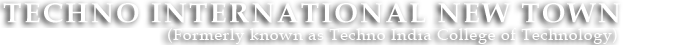#+91 9674112076/2079
••••# Microprocessor Lab

MICROPROCESSOR & MICROCONTROLLER LABORATORY

Code: EC 592, CS 592, EE 594C

List of Experiments:
1. WAP to transfer an 8 bit data from register B to accumulator.
2. WAP to transfer an 8 bit data from memory location to accumulator.
3. WAP to transfer the data byte to accumulator by using 1) MOV, 2) LDA, 3) LDAX.
4. WAP to find out the 2’s complement of a given 8 bit number.
5. WAP to find out the 2’s complement of a given 16 bit number.
6. WAP to find out the numbers of odd and even numbers from an array of numbers.
7. WAP to add two 8 bit numbers considering the carry.
8. WAP to subtract two 16 bit numbers considering the borrow.
9. WAP to mask the lower nibble of an 8 bit numbers.
10. WAP to shift an 8 bit number left by 2 bit.
11. WAP to shift an 16 bit number by 2 bit without using rotate instruction
12. WAP to find out the square of an 8 bit using look up table.
13. Find out the smallest and largest from a array of numbers.
14. Find out the sum of a series of 8 bit numbers.
15. Find out the smallest and largest from a array of numbers.
16. Find out the sum of a series of 8 bit numbers.
17. Sort array of numbers in ascending and descending order.
18. WAP to multiply two 8 bit numbers.
19. Transfer block of N-bytes from source to destination in forward and reverse order.
20. Unpack a BCD number.
21. Pack two BCD numbers.
22. WAP to convert two BCD numbers in memory location to the equivalent HEX number.
23. WAP to find out the factorial of a given number.
24. WAP to interface 8255A with 8085 or 8086 microprocessor kit.
25. Study of 8251 microcontroller kit and Interfacing of keyboard with 8251 microcontroller